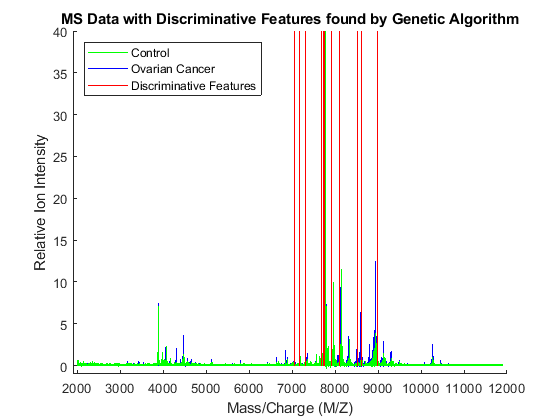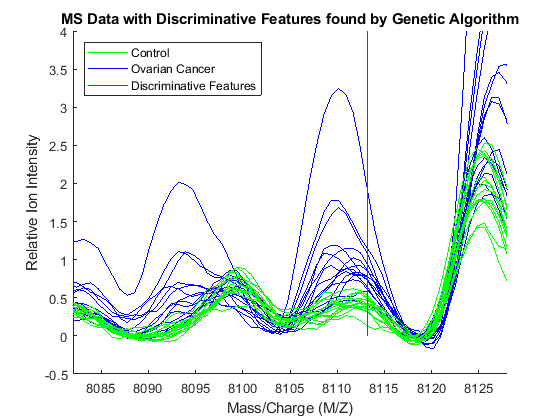# Genetic Algorithm Search for Features in Mass Spectrometry Data

This example shows how to use the Global Optimization Toolbox with the Bioinformatics Toolbox™ to optimize the search for features to classify mass spectrometry (SELDI) data.

### Introduction

Genetic algorithms optimize search results for problems with large data sets. You can use the MATLAB® genetic algorithm function to solve these problems in Bioinformatics. Genetic algorithms have been applied to phylogenetic tree building, gene expression and mass spectrometry data analysis, and many other areas of Bioinformatics that have large and computationally expensive problems. This example searches for optimal features (peaks) in mass spectrometry data. We will look for specific peaks in the data that distinguish cancer patients from control patients.

### Global Optimization Toolbox

First familiarize yourself with the Global Optimization Toolbox. The documentation describes how a genetic algorithm works and how to use it in MATLAB. To access the documentation, use the doc command.

```doc ga ```

### Preprocess Mass Spectrometry Data

The data in this example is available from the FDA-NCI Clinical Proteomics Program Databank at http://home.ccr.cancer.gov/ncifdaproteomics/ppatterns.asp. It is a collection of samples from 121 ovarian cancer patients and 95 control patients.

This example assumes that you already have the preprocessed data `OvarianCancerQAQCdataset.mat`. However, if you do not have the data file, you can recreate by following the steps in the example Batch Processing of Spectra Using Sequential and Parallel Computing.

Alternatively, you can run the script `msseqprocessing.m`.

```addpath(fullfile(matlabroot,'examples','bioinfo','main')) % Make sure the supporting files are on the search path type msseqprocessing ```
```% MSSEQPROCESSING Script to create OvarianCancerQAQCdataset.mat (used in % CANCERDETECTDEMO). Before running this file initialize the variable % "repository" to the full path where you placed you mass-spectrometry % files. For Example: % % repository = 'F:/MassSpecRepository/OvarianCD_PostQAQC/'; % % or % % repository = '/home/username/MassSpecRepository/OvarianCD_PostQAQC/'; % % The approximate time of execution is 18 minutes (Pentium 4, 4GHz). If you % have the Parallel Computing Toolbox refer to BIODISTCOMPDEMO to see % how you can speed this analysis up. % Copyright 2003-2008 The MathWorks, Inc. repositoryC = [repository 'Cancer/']; repositoryN = [repository 'Normal/']; filesCancer = dir([repositoryC '*.txt']); NumberCancerDatasets = numel(filesCancer); fprintf('Found %d Cancer mass-spectrograms.\n',NumberCancerDatasets) filesNormal = dir([repositoryN '*.txt']); NumberNormalDatasets = numel(filesNormal); fprintf('Found %d Control mass-spectrograms.\n',NumberNormalDatasets) files = [ strcat('Cancer/',{filesCancer.name}) ... strcat('Normal/',{filesNormal.name})]; N = numel(files); % total number of files fprintf('Total %d mass-spectrograms to process...\n',N) [MZ,Y] = msbatchprocessing(repository,files); disp('Finished; normalizing and saving to OvarianCancerQAQCdataset.mat.') Y = msnorm(MZ,Y,'QUANTILE',0.5,'LIMITS',[3500 11000],'MAX',50); grp = [repmat({'Cancer'},size(filesCancer));... repmat({'Normal'},size(filesNormal))]; save OvarianCancerQAQCdataset.mat Y MZ grp ```

### Load Mass Spectrometry Data into MATLAB®

Once you have the preprocessed data, you can load it into MATLAB.

```load OvarianCancerQAQCdataset whos ```
``` Name Size Bytes Class Attributes MZ 15000x1 120000 double Y 15000x216 25920000 double grp 216x1 25056 cell ```

There are three variables: MZ, Y, grp. MZ is the mass/charge vector, Y is the intensity values for all 216 patients (control and cancer), and grp holds the index information as to which of these samples represent cancer patients and which ones represent normal patients. To visualize this data, see the example Identifying Significant Features and Classifying Protein Profiles.

Initialize the variables used in the example.

```[numPoints, numSamples] = size(Y); % total number of samples and data points id = grp2idx(grp); % ground truth: Cancer=1, Control=2 ```

### Create a Fitness Function for the Genetic Algorithm

A genetic algorithm requires an objective function, also known as the fitness function, which describes the phenomenon that we want to optimize. In this example, the genetic algorithm machinery tests small subsets of M/Z values using the fitness function and then determines which M/Z values get passed on to or removed from each subsequent generation. The fitness function biogafit is passed to the genetic algorithm solver using a function handle. In this example, biogafit maximizes the separability of two classes by using a linear combination of 1) the a-posteriori probability and 2) the empirical error rate of a linear classifier (classify). You can create your own fitness function to try different classifiers or alternative methods for assessing the performance of the classifiers.

```type biogafit ```
```function classPerformance = biogafit(thePopulation,Y,id) %BIOGAFIT The fitness function for BIOGAMSDEMO % % This function uses the classify function to measure how well mass % spectrometry data is grouped using certain masses. The input argument % thePopulation is a vector of row indices from the mass spectrometry % data Y. Classification performance is a linear combination of the error % rate and the posteriori probability of the classifier. % Copyright 2003-2013 The MathWorks, Inc. thePopulation = round(thePopulation); try [c,~,p] = classify(Y(thePopulation,:)',Y(thePopulation,:)',double(id),'linear'); cp = classperf(id,c); classPerformance = 100*cp.ErrorRate + 1 - mean(max(p,[],2)); catch % In case pooled covariance matrix is not positive definite we try a % naive-Bayes classifier: try [c,~,p] = classify(Y(thePopulation,:)',Y(thePopulation,:)',double(id),'diaglinear'); cp = classperf(id,c); classPerformance = 100*cp.ErrorRate + 1 - mean(max(p,[],2)); catch classPerformance = Inf; end end ```

### Create an Initial Population

Users can change how the optimization is performed by the genetic algorithm by creating custom functions for crossover, fitness scaling, mutation, selection, and population creation. In this example you will use the biogacreate function written for this example to create initial random data points from the mass spectrometry data. The function header requires specific input parameters as specified by the GA documentation. There is a default creation function in the toolbox for creating initial populations of data points.

```type biogacreate ```
```function pop = biogacreate(GenomeLength,~,options,Y,id) %BIOGACREATE Population creation function for MSGADEMO % % This function creates a population matrix with dimensions of % options.PopulationSize rows by the number of independent variables % (GenomeLength) columns. These values are integers that correspond to % randomly selected rows of the mass spectrometry data Y. Each row of the % population matrix is a random sample of row indices of the mass spec % data. % Note: This function's input arguments are required by the GA function. % See GA documentation for further detail. % Copyright 2005-2013 The MathWorks, Inc. pop = zeros(options.PopulationSize,GenomeLength); npop = numel(pop); ranked_features = rankfeatures(Y,id,'NumberOfIndices',npop,'NWeighting',.5); pop(:) = randsample(ranked_features,npop); ```

### Set Genetic Algorithm Options

The GA function uses an options structure to hold the algorithm parameters that it uses when performing a minimization with a genetic algorithm. The optimoptions function will create this options structure. For the purposes of this example, the genetic algorithm will run only for 50 generations. However, you may set 'Generations' to a larger value.

```options = optimoptions('ga','CreationFcn',{@biogacreate,Y,id},... 'PopulationSize',120,... 'Generations',50,... 'Display','iter') ```
```options = ga options: Set properties: CreationFcn: {1x3 cell} Display: 'iter' MaxGenerations: 50 PopulationSize: 120 Default properties: ConstraintTolerance: 1.0000e-03 CrossoverFcn: @crossoverscattered CrossoverFraction: 0.8000 EliteCount: '0.05*PopulationSize' FitnessLimit: -Inf FitnessScalingFcn: @fitscalingrank FunctionTolerance: 1.0000e-06 HybridFcn: [] InitialPopulationMatrix: [] InitialPopulationRange: [] InitialScoresMatrix: [] MaxStallGenerations: 50 MaxStallTime: Inf MaxTime: Inf MutationFcn: {@mutationgaussian  } NonlinearConstraintAlgorithm: 'auglag' OutputFcn: [] PlotFcn: [] PopulationType: 'doubleVector' SelectionFcn: @selectionstochunif UseParallel: 0 UseVectorized: 0 ```

### Run GA to Find 20 Discriminative Features

Use ga to start the genetic algorithm function. 100 groups of 20 datapoints each will evolve over 50 generations. Selection, crossover, and mutation events generate a new population in every generation.

```% initialize the random generators to the same state used to generate the % published example rng('default') nVars = 12; % set the number of desired features FitnessFcn = {@biogafit,Y,id}; % set the fitness function feat = ga(FitnessFcn,nVars,options); % call the Genetic Algorithm feat = round(feat); Significant_Masses = MZ(feat) cp = classperf(classify(Y(feat,:)',Y(feat,:)',id),id); cp.CorrectRate ```
``` Best Mean Stall Generation Func-count f(x) f(x) Generations 1 240 2.827 8.928 0 2 354 2.827 8.718 1 3 468 0.9663 8.001 0 4 582 0.9516 7.249 0 5 696 0.9516 6.903 1 6 810 0.4926 6.804 0 7 924 0.4926 6.301 1 8 1038 0.02443 5.215 0 9 1152 0.02443 4.77 1 10 1266 0.02101 4.084 0 11 1380 0.02101 3.792 1 12 1494 0.01854 3.437 0 13 1608 0.01606 3.44 0 14 1722 0.01372 2.768 0 15 1836 0.01218 2.74 0 16 1950 0.01204 2.471 0 17 2064 0.01204 2.649 1 18 2178 0.01189 2.326 0 19 2292 0.01189 2.003 0 20 2406 0.0118 2.341 0 21 2520 0.01099 1.714 0 22 2634 0.01094 1.828 0 23 2748 0.01094 1.94 1 24 2862 0.01094 2.285 2 25 2976 0.009843 2.026 0 26 3090 0.009843 1.899 1 27 3204 0.009183 1.802 0 28 3318 0.007877 1.5 0 29 3432 0.007788 1.793 0 30 3546 0.007788 1.756 1 Best Mean Stall Generation Func-count f(x) f(x) Generations 31 3660 0.007091 1.719 0 32 3774 0.006982 1.598 0 33 3888 0.006982 1.269 1 34 4002 0.006732 1.279 0 35 4116 0.005008 1.229 0 36 4230 0.004325 1.179 0 37 4344 0.004325 1.534 1 38 4458 0.003982 1.15 0 39 4572 0.003982 0.9602 1 40 4686 0.003982 0.8547 2 41 4800 0.003891 0.9083 0 42 4914 0.003683 0.7409 0 43 5028 0.003683 0.516 1 44 5142 0.003364 0.5153 0 45 5256 0.003172 0.4218 0 46 5370 0.003172 0.3783 1 47 5484 0.002997 0.1883 0 48 5598 0.002675 0.1297 0 49 5712 0.002611 0.04382 0 50 5826 0.002519 0.007859 0 Optimization terminated: maximum number of generations exceeded. Significant_Masses = 1.0e+03 * 7.6861 7.9234 8.9834 8.6171 7.1808 7.3057 8.1132 8.5241 7.0527 7.7600 7.7442 7.7245 ans = 1 ```

### Display the Features that are Discriminatory

To visualize which features have been selected by the genetic algorithm, the data is plotted with peak positions marked with red vertical lines.

```xAxisLabel = 'Mass/Charge (M/Z)'; % x label for plots yAxisLabel = 'Relative Ion Intensity'; % y label for plots figure; hold on; hC = plot(MZ,Y(:,1:15) ,'b'); hN = plot(MZ,Y(:,141:155),'g'); hG = plot(MZ(feat(ceil((1:3*nVars )/3))), repmat([0 100 NaN],1,nVars),'r'); xlabel(xAxisLabel); ylabel(yAxisLabel); axis([1900 12000 -1 40]); legend([hN(1),hC(1),hG(1)],{'Control','Ovarian Cancer', 'Discriminative Features'}, ... 'Location', 'NorthWest'); title('MS Data with Discriminative Features found by Genetic Algorithm'); ```Observe the interesting peak around 8100 Da., which seems to be shifted to the right on healthy samples.

```axis([8082 8128 -.5 4]) ```##### SupportGet trial now